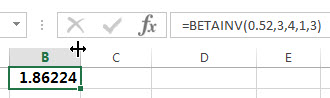# Excel BETAINV Function

This post will guide you how to use the BETAINV function with syntax and examples in Microsoft excel.

### Description

The Excel BETAINV function returns the inverse of the beta cumulative probability density function. So if the probability is equal to BETADIST(x,…), then the BETAINV(probability,…) will return x. and this function has been replaced by the new function BETA.INV function.

The BETAINV function is a build-in function in Microsoft Excel and it is categorized as a Statistical Function.

The BETAINV function is available in Excel 2016, Excel 2013, Excel 2010, Excel 2007, Excel 2003, Excel XP, Excel 2000, Excel 2011 for Mac.

### Syntax

The syntax of the BETAINV function is as below:

`= BETAINV(probability, alpha, beta, [A], [B])`

Where the BETAINV function arguments are:

• Probability -This is a required argument.  A probability associated with the beta distribution, for which you wish to find the value of x.
• Alpha – This is a required argument.  It is a parameter of the distribution.
• Beta – This is a required argument.  It is a parameter of the distribution.
• A – This is an optional argument. A lower bound to the interval of x. If it is omitted, it will be set the default value 0.
• B – This is an optional argument. A upper bound to the interval of x. If it is omitted, it will be set the default value 1.

Note:

• If any argument is non-numeric, the BETAINV function will return the #VALUE! Error.
• If the Alpha or Beta argument is smaller than or equal to 0, then the BETAINV function will return #NUM! Error.
• If the argument probability is small than or equal to 0 or greater than 1, the BETAINV function will Return #NUM! Error.

### Excel BETAINV Function Examples

The below examples will show you how to use Excel BETAINV Function to calculate the cumulative beta distribution or beta probability density function.

1# to calculate the inverse of the cumulative beta probability density function for a supplied set of parameters, using the following formula:

`=BETAINV(0.52,3,4,1,3)`### Related Functions

• Excel BETA.DIST function
The Excel BETA.DIST function can be used to calculate the cumulative beta distribution or beta probability density function.The syntax of the BETA.DIST function is as below:= BETA.DIST(x, alpha, beta, cumulative, [A], [B]).…
The Excel BETADIST function returns the cumulative beta probability density function.The syntax of the BETADIST function is as below:= BETADIST(x, alpha, beta, [A], [B]).…
• Excel BETA.INV function
The Excel BETA.INV function returns the inverse of the beta cumulative probability density function. So if the probability is equal to BETA.DIST(x,…TRUE), then the BETA.INV(probability,…) will return x.The syntax of the BETA.INV function is as below:= BETA.INV(probability, alpha, beta, [A], [B]).…
• Excel BINOM.DIST function
The Excel BINOM.DIST function returns the individual term binomial distribution probability. The syntax of the BINOM.DIST function is as below:= BINOM.DIST(number_s, trials, probability_s, cumulative).…
Related Posts

Excel DVARP Function

This post will guide you how to use Excel DVARP function with syntax and examples in Microsoft excel. Description The Excel DVARP Function will get the variance of a population based on the entire population of numbers in a column ...

Excel DVAR Function

This post will guide you how to use Excel DVAR function with syntax and examples in Microsoft excel. Description The Excel DVAR Function will get the variance of a population based on a sample of numbers in a column in ...

Excel DSUM Function

This post will guide you how to use Excel DSUM function with syntax and examples in Microsoft excel. Description The Excel DSUM Function will add the numbers in a column or database that meets a given criteria. And so you ...

Excel DSTDEVP Function

This post will guide you how to use Excel DSTDEVP function with syntax and examples in Microsoft excel. Description The Excel DSTDEVP returns the standard deviation of a population based on the entire population of numbers in a column or ...

Excel DSTDEV Function

This post will guide you how to use Excel DSTDEV function with syntax and examples in Microsoft excel. Description The Excel DSTDEV returns the standard deviation of a population based on a sample of numbers in a column or database ...

Excel DMIN Function

This post will guide you how to use Excel DMIN function with syntax and examples in Microsoft excel. Description The Excel DMIN returns the minimum value from a database or a column of a list that matches the specified conditions. ...

Excel DPRODUCT Function

This post will guide you how to use Excel DPRODUCT function with syntax and examples in Microsoft excel. Description The Excel DPRODUCT returns the product of values from a set of records that match criteria that you specify. The DPRODUCT ...

Excel XIRR Function

This post will guide you how to use Excel XIRR function with syntax and examples in Microsoft excel. Description The Excel XIRR function returns the internal rate of return for a series of cash flows that is not necessarily periodic. ...

Excel VDB Function

This post will guide you how to use Excel VDB function with syntax and examples in Microsoft excel. Description The Excel VDB function calculates the depreciation of an asset for a specified period based on the double declining balance method. ...

Excel SYD Function

This post will guide you how to use Excel SYD function with syntax and examples in Microsoft excel. Description The Excel SYD function calculates the sum-of-years’digits depreciation of an asset for a specified period. And it will return a numeric ...

Sidebar